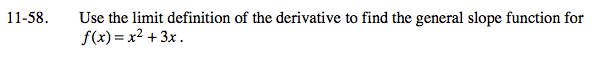Home > PC > Chapter 11 > Lesson 11.1.4 > Problem11-58

11-58.

Use the limit definition of the derivative to find the general slope function for f(x) = x2 + 3x. Homework Help ✎$\textit{f}'(\textit{x})=\lim_{ \textit{h} \to 0 }\frac{\textit{f}(\textit{x}+\textit{h})-\textit{f}(\textit{x})}{\textit{h}$

$\textit{f}'(\textit{x})=\lim_{ \textit{h} \to 0 }\frac{((\textit{x}+\textit{h})^2+3(\textit{x}+\textit{h}))-(\textit{x}^2+3\textit{x})}{\textit{h}$

Now simplify and to find the limit.